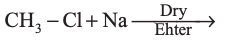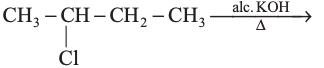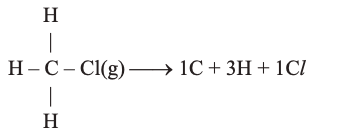### CBSE Class 11 Chemistry Sample Paper 4# CBSE CLASS 11 CHEMISTRY SAMPLE PAPER – 4

CBSE Board

Class 11th Chemistry

Sample Paper 4

Time: 3 Hrs                                                                                                                     Total Marks: 70

General Instructions

• All questions are compulsory.
• Q. No. 1 to 5 are Very Short Answer Question carrying 1 mark each.
• Q. No. 6 to 12 are Short Answer Questions and carrying 2 marks each.
• Q. No. 13 to 24 are Short Answer Questions and carrying 3 marks each.
• Q. No. 25 to 27 are Long Answer Questions and carrying 5 marks each.
• Use log tables, if necessary, use of calculator is not allowed.

 Q.No. Question Marks 1. Define electron gain enthalpy. 1 2. Write I.U.P.A.C. name of the following compound:1 3. Define standad enthalpy of formation. 1 4. Write electronic configuration of $mathrm{Cu}^{2+}$ ion. (Atomic number of $mathrm{Cu}=29$ ). 1 5. In which orbital will the electrons enter first 3 d or 4p ? 1 6. Give the units of vander waal’s constants. Also point out their significance. 2 7. $0.3780 mathrm{~g}$ of an organic chloro compound gave $0.5740 mathrm{~ g}$ of silver chloride in carius estimation. Calculate the percentage of chlorine present in compound. 2 8. Write the short notes on: 2 (a) Wurtz Reaction (b) Freidal Craft’s Alkylation 9. Write the molecular shapes of: 2 (a) $mathrm{XeF}_{4}$ (b) $mathrm{CIF}_{3}$ 10. Determine the emperical formula of an oxide of iron which has $69.9 %$ iron and $30.1 %$ dioxygen by man. $quad$ [Atomic mass: $mathrm{Fe}=55.85, mathrm{O}=16.00]$ 2 11. The ionization enthalpy of lithium is $520 mathrm{kJmol}^{-1}$, calculate the amount of energy required to convert $140 mathrm{mg}$ of lithium atoms in gaseous state into $mathrm{Li}^{+}$ ion. 2 12. How does Heisenberg’s uncertanity principle support concept of orbital? 2 13. Compelete the following reactions: 3 (a)(b)(c)14. Balance the following reaction in acidic medium: $mathrm{MnO}_{4}^{-}(mathrm{aq})+mathrm{SO}_{2}(mathrm{~g}) longrightarrow mathrm{Mn}^{2+}(mathrm{aq})+mathrm{HSO}_{4}^{-}(mathrm{aq})$ 3 15. The value of $mathrm{K}_{mathrm{C}}$ for the reaction: $2 mathrm{~A} rightleftharpoons mathrm{B}+mathrm{C}$ is $2 times 10^{-3}$ at $500 mathrm{~K}$. At given time, the composition of reaction mixture is $[mathrm{A}]=[mathrm{B}]=[mathrm{C}]=3 times 10^{-4} mathrm{M}$. Is the reaction mixture at equilibrium? If not, what is the direction of net reaction? 3 16. Write down the nature of below reaction with reason: 3 (a) $mathrm{NH}_{3}+mathrm{H}^{+} rightarrow mathrm{NH}_{4}^{+}$ (b) $mathrm{BF}_{3}+mathrm{NH}_{3} rightarrow mathrm{F}_{3} mathrm{~B} leftarrow mathrm{NH}_{3}$ (c) $mathrm{H}_{2} mathrm{SO}_{4} rightarrow mathrm{H}^{+}+mathrm{HSO}_{4}^{-}$ 17. Arrange the following carbocation in creasing order of their stability. $left(mathrm{CH}_{3}right)_{2} mathrm{CH}^{+}, mathrm{CH}_{3} mathrm{CH}_{2}^{+},left(mathrm{CH}_{3}right)_{3} mathrm{C}^{+}, mathrm{CH}_{3}^{+}$ 3 18. How will you convert 3 (a) Propan-1-ol into propene (b) 2-bromopropane into But-2-ene (c) Ethyl amine into ethyl isocyanide 19. 3 (a) Define eutrophication and penumocanosis. (b) Write difference in between photochemical and classical smog. 20. 3 (a) Calculate the oxidation number of $mathrm{S}$ in $mathrm{S}_{2} mathrm{O}_{6}^{-2}$ having $(-mathrm{O}-mathrm{O}-)^{2-}$ linkage and $mathrm{C}$ in $mathrm{CH}_{3} mathrm{COOH}$. (b) Balance the equation in basic medium by half reaction method $$mathbf{P}_{4}(s) rightarrow P H_{3}(g)+H_{2} P O_{2}^{-}(A q)$$ 21. 3 (a) Out of staggerd and eclipsed conformations of n-butane, which is more stable and why? (b) What causes the temporary and permanent hardness of water. 22. Write a breif note on the following environmental terms: 3 (a) Acid rains (b) Eutrophication/Green House effect (c) Green chemistry 23. 3 (a) Define buffer solution. (b) The solubility of $mathrm{Sr}(mathrm{OH})_{2}$ at $298 mathrm{~K}$ is $19.23 mathrm{~g} / mathrm{L}$ of solution. Calculate the concentration of strontium and hydroxyl ions and the $mathrm{pH}$ of the solution. 24. Write any three main biological importance of $mathrm{Ca}$ and $mathrm{Mg}$. 3 25. 5 (a) The stability of peroxide and superoxide of alkali metals increases as we go down the group. Explain giving reasons. (b) How to control photochemical smog. OR (a) Derive first law of thermodynamics. (b) Define enthalpy of neutralisation. (c) Calculate the $Delta mathrm{H}^{theta}$ of the reaction.Bone enthalpies of $mathrm{C}-mathrm{H} & mathrm{C}-mathrm{Cl}$ bond are $415 mathrm{~kJ} mathrm{~mol}^{-1} & 326 mathrm{~kJ} mathrm{~mol}^{-1}$ 26. 5 (a) Account for the following: i. Boron Halides do not dimerise like $mathrm{BH}_{3}$. ii. Carbon shows catenation iii. $mathrm{PbCl}_{4}$ is a good oxidising agent. (b) Complete the following reactions: i. $mathrm{B}_{2} mathrm{H}_{6}+3 mathrm{O}_{2} longrightarrow$ ii. $2 mathrm{BF}_{3}+6 mathrm{NaH} stackrel{450 mathrm{~K}}{longrightarrow}$ OR (a) Write equation to justify amphoteric nature of Water. (b) What is application of equilibrium constant. (c) What are the full form of BOD? 27. 5 (a) Is the entropy of the universe constant? (b) If standard free energy change for a reaction is found to be zero, what is its equilibrium constant. (c) Define common ion effect. OR (a) Calculate the degree of ionisation of $0.1 mathrm{~mol} / mathrm{L}$ solution of acetic acid, given $mathrm{K}_{mathrm{a}}$ for $mathrm{CH}_{3} mathrm{COOH} 1.8 times 10^{-5} mathrm{~mol} / mathrm{L}$ (b) Define the following terms : i. Solubility product ii. Buffer solution iii. Henderson equation
Privacy Settings